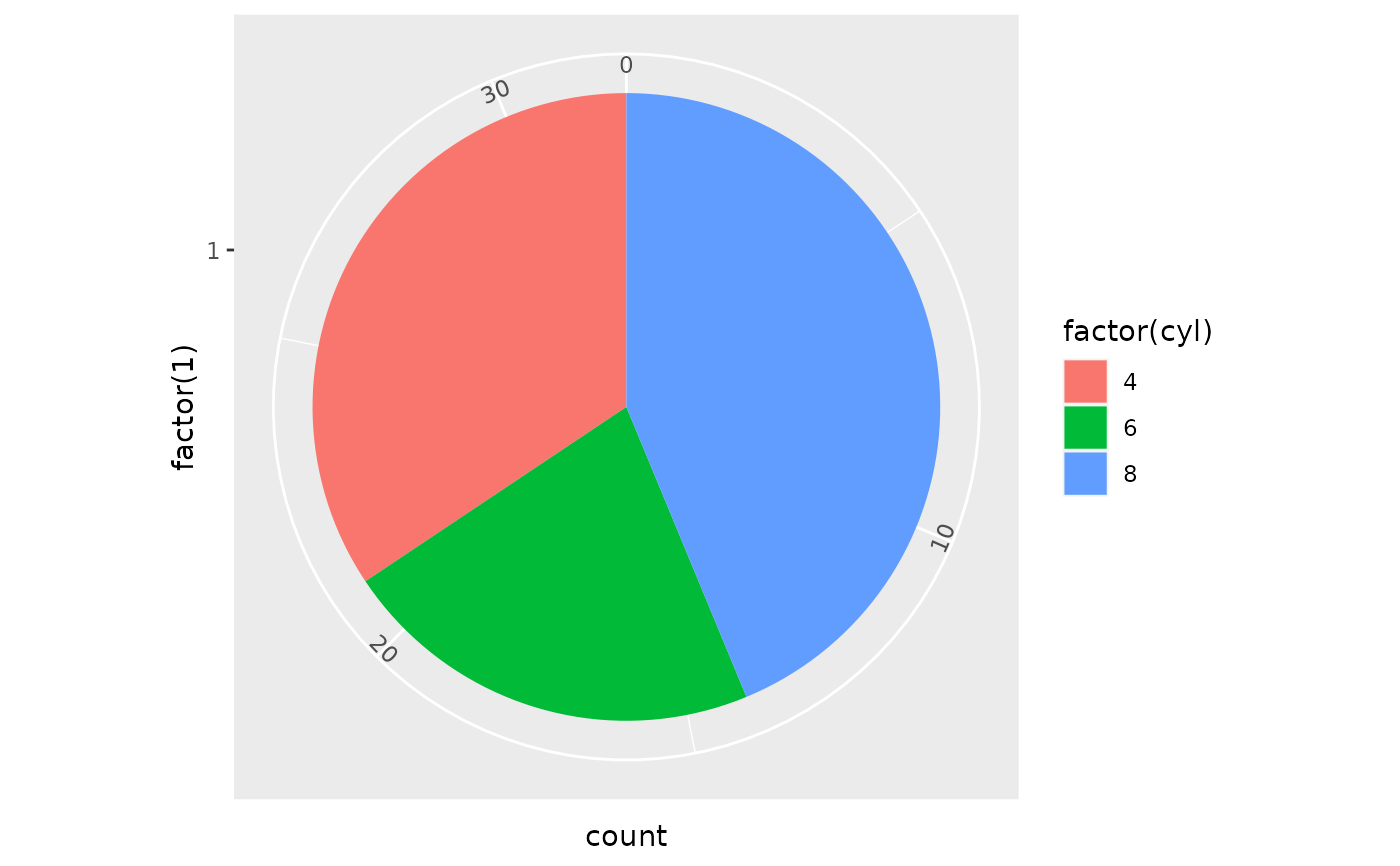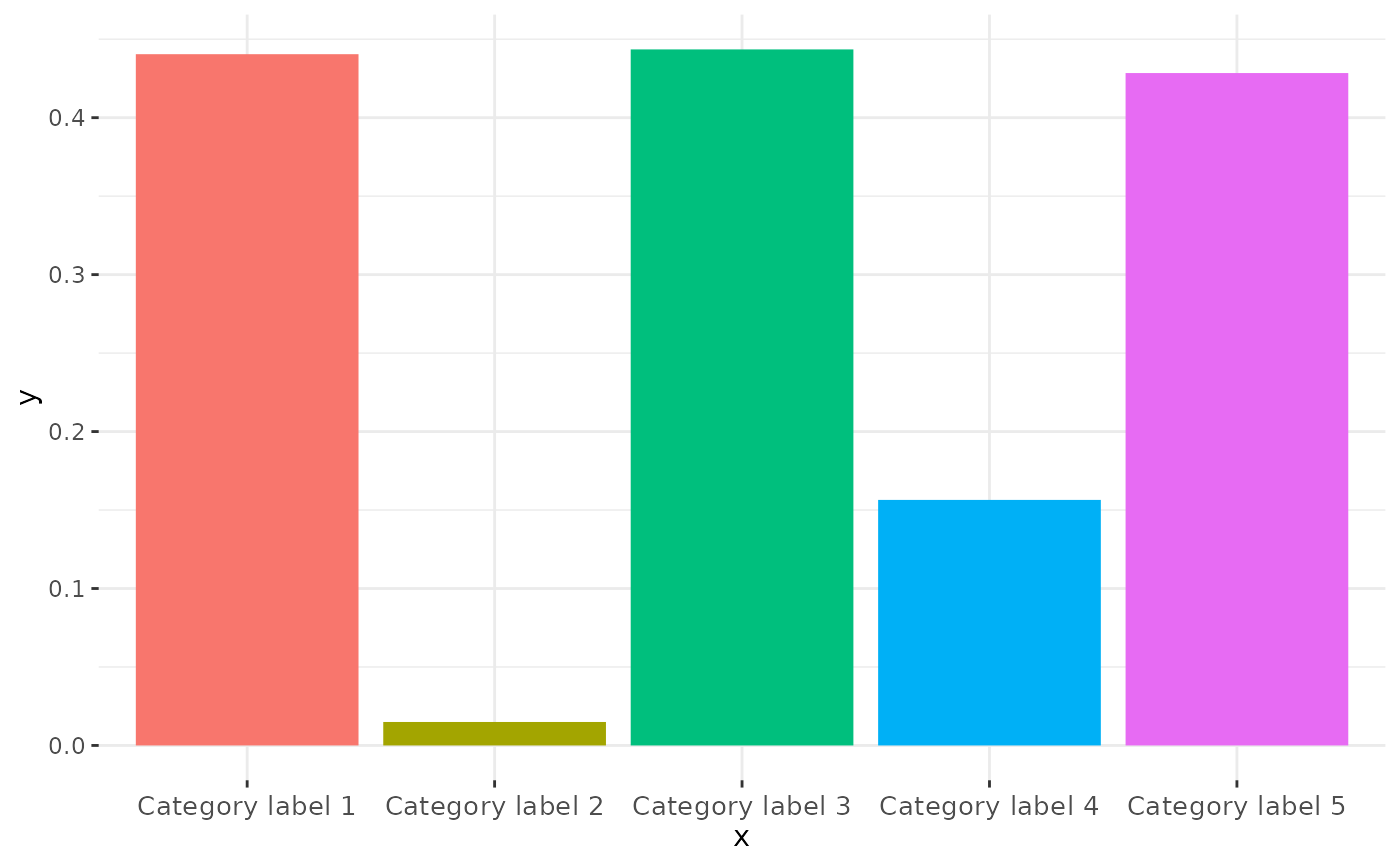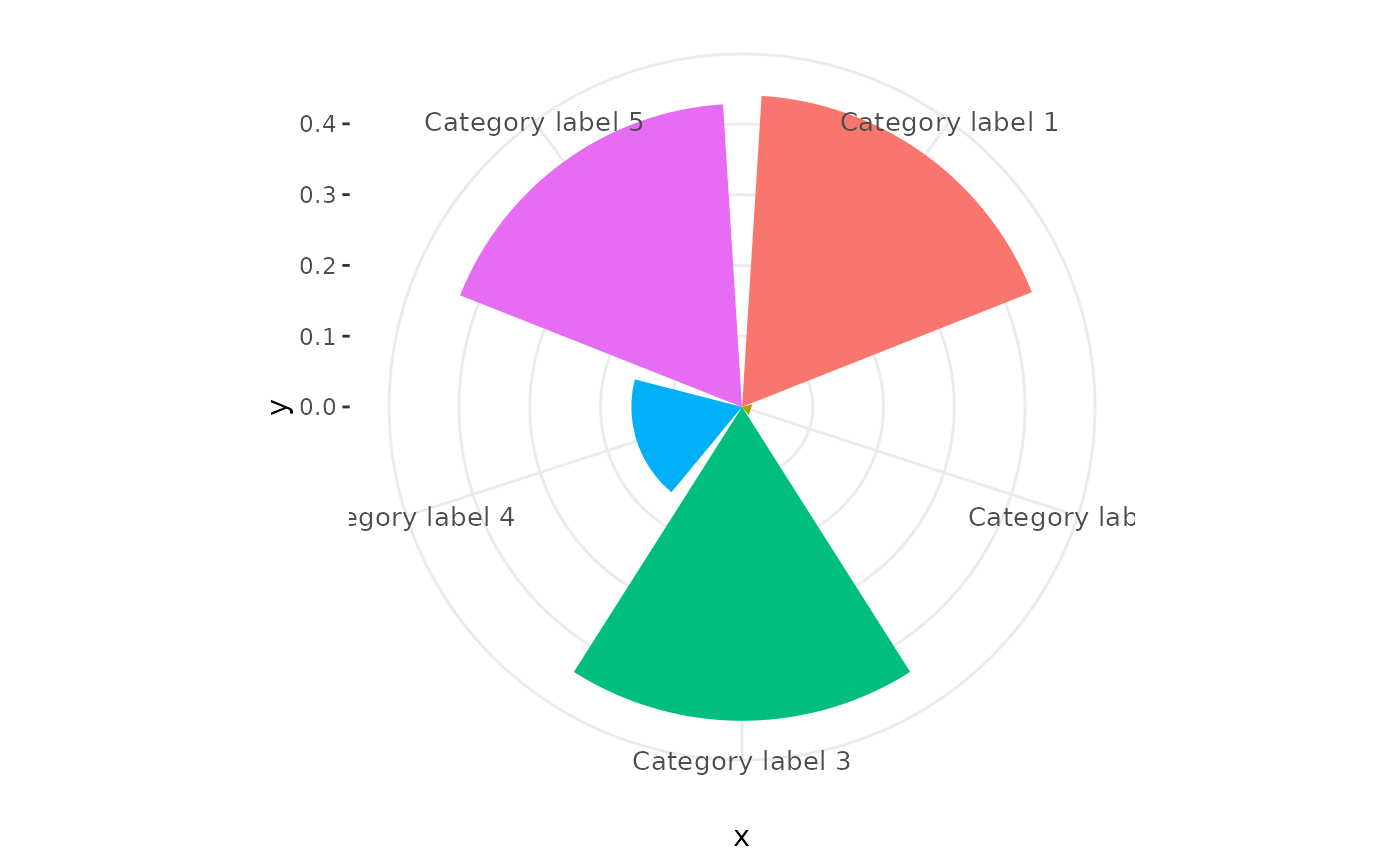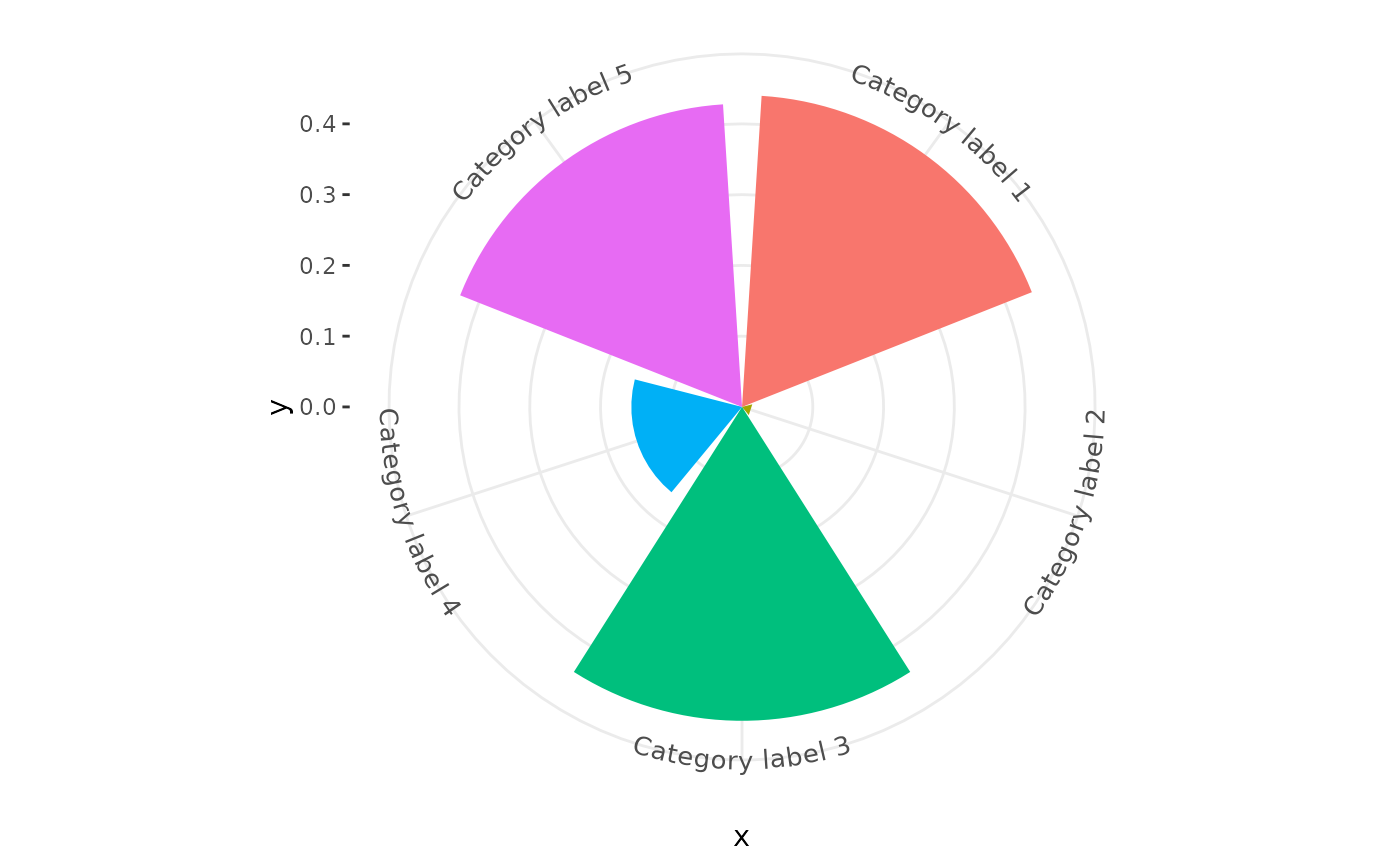Polar co-ordinates in ggplot2 help to create a range of circular plots, which can be used to present data in a visually appealing, user-friendly way. However, the standard coord_polar uses a textGrob to render the labels on the circumferential (theta) axis, meaning that labels do not rotate or curve in line with the axis. coord_curvedpolar aims to be identical to coord_polar, except that the text on the theta axis follows the curve of the plot, correcting automatically for resizing to preserve letter spacing and size.

## Usage

coord_curvedpolar(
theta = "x",
start = 0,
direction = 1,
clip = "on",
halign = c("center")
)

## Arguments

theta

variable to map angle to (x or y)

start

Offset of starting point from 12 o'clock in radians. Offset is applied clockwise or anticlockwise depending on value of direction.

direction

1, clockwise; -1, anticlockwise

clip

Should drawing be clipped to the extent of the plot panel? A setting of "on" (the default) means yes, and a setting of "off" means no. For details, please see [coord_cartesian()].

halign

A character(1) describing how multi-line text should be justified. Can either be "center" (default), "left" or "right".

## Value

A Coord ggproto object that can be added to a plot.

## Examples


# A pie chart = stacked bar chart + polar coordinates
pie <- ggplot(mtcars, aes(x = factor(1), fill = factor(cyl))) +
geom_bar(width = 1)
pie + coord_curvedpolar(theta = "y")# Demonstrating curved category labels
p <- ggplot(data.frame(x = paste("Category label", 1:5), y = runif(5)),
aes(x, y, fill = x)) +
geom_col() +
theme_bw() +
theme(panel.border = element_blank(),
legend.position = "none",
axis.text.x = element_text(size = 10, vjust = 0.5))

# Standard bar chart in Cartesian Co-ordinates
p# Standard coord_polar axis labels
p + coord_polar()# Curved polar co-ordinate labels
p + coord_curvedpolar()